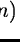Next: Order Definition Up: Echelle Reduction Method Previous: Retrieving demonstration and calibration

General Description

The first problem in the reduction of echelle spectra is, of course, the solution of the dispersion relation. That is the mapping between the spacewavelength, spectral order and the space (x,y) sample x, line y in the raw image. This relation gives the position of the orders on the raw image, and defines the wavelength scale of the extracted spectrum. The mapping is performed in two steps:
• A first operation (order definition), gives the position of the orders in the raw image. In figure 7.1, this operation corresponds to the step Find Order Position''. The required input is an order reference frame (usually FLAT or STD) and the output is a set of polynomial coefficients. These coefficients are an input of the step Extract Orders''.
• A second operation (wavelength calibration) defines the wavelength scale of the extracted spectrum. The successive steps of this operation are shown in the second column of figure 7.1. The output is a set of dispersion coefficients required by the step Sample in Wavelength''.
Sections 7.2 and 7.6 describe the solution of this mapping.

The second step in the reduction, described in Section 7.4, is to estimate the image background. The background depends mainly on the characteristics of the detector, but includes the additional components of the scattered light in the optics and spectrograph. This operation corresponds to the step Subtract Background'' in fig. 7.1.

A particular problem in the CCD-detector used by the two echelle instruments is the appearence of interference fringes produced within the silicon, which can be especially important in the long wavelength range of the instrument. By processing the flat-field (first column of fig. 7.1), correction frames are prepared and used for the standard star and the object reduction. A method to correct for this effect is described in Section 7.7.

After the corrections for all these effects, the information in the spectral orders is extracted using the methods described in Section 7.5. The extracted flux, used in conjunction with the dispersion relation, gives the photometric profiles of the spectral orders. Two instrumental effects are still present in these profiles: first, due to the blaze effect of the echelle grating, the efficiency of the spectrograph changes along each order; second, the efficiency of the whole instrument is not uniform with wavelength. In Section 7.8 we describe how to correct both effects, to normalize the fluxes and, if the input data includes calibration stars, to convert the fluxes into absolute units.

Note
Taking a standard star exposure (STD) is a recommended observation strategy which can make easier the order definition in the blue part of the spectrum as well as the correction of individual orders for the variations of grating efficiency (blaze function).

The steps summarised above comprise the STANDARD reduction. Alternatively, it is possible to correct the variation in sensitivity along the spectral orders using a suitable model for the blaze function as described in Section 7.8.2. Figure 7.1 displays the process scheme in a typical reduction session; slanted fonts indicate optional operations. In the rest of this Section the algorithms used in each step of the reduction are described.Next: Order Definition Up: Echelle Reduction Method Previous: Retrieving demonstration and calibration
Petra Nass
1999-06-15